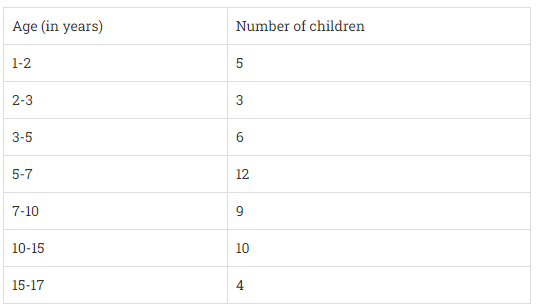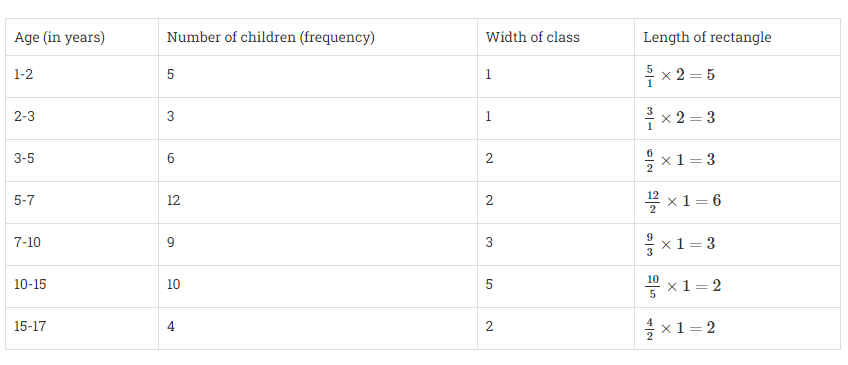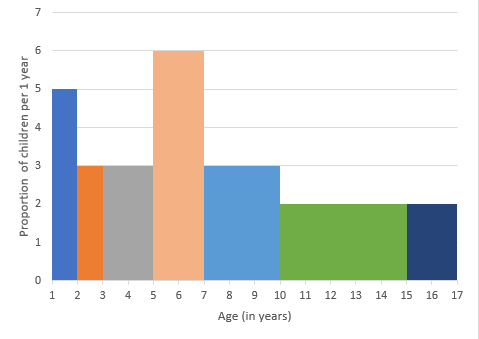Draw a histogram to represent the data above."
">

# A random survey of the number of children of various age groups playing in a park was found as follows:Draw a histogram to represent the data above."

Given:

A random survey of the number of children of various age groups playing in a park.

To do:

We have to draw a histogram to represent the data above.

Solution:

The width of the class intervals in the given data is varying.

We know that,

The area of rectangle is proportional to the frequencies in the histogram.

This implies,

The proportion of the children per year can be calculated as given in the below table:Let the x-axis represent the age of children and the y-axis represent the proportion of children per 1-year interval.

The required histogram is as follows:Updated on: 10-Oct-2022

52 Views